Ex 12.3

Chapter 12 Class 10 Areas related to Circles
Serial order wise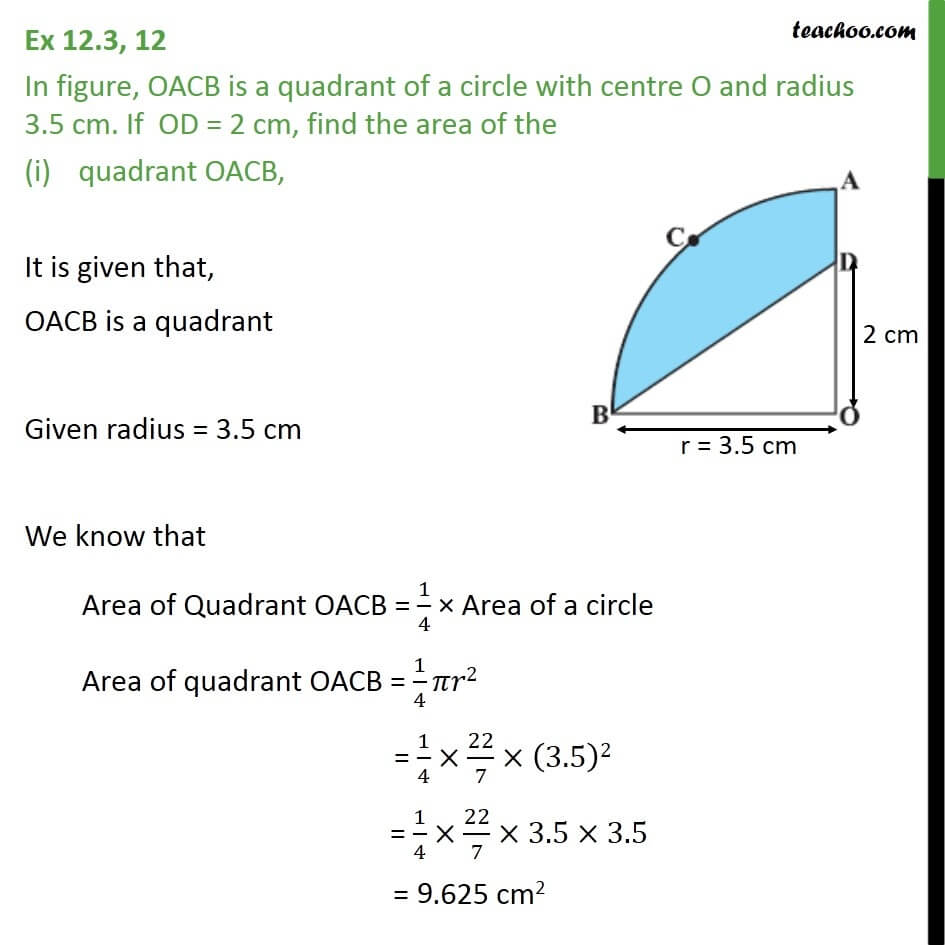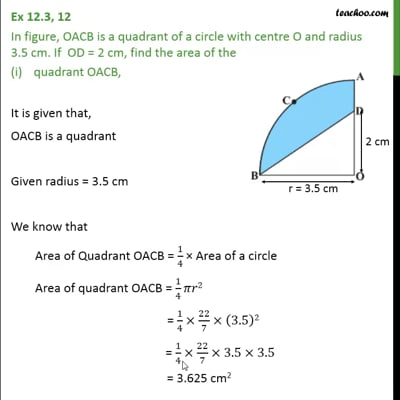This video is only available for Teachoo black users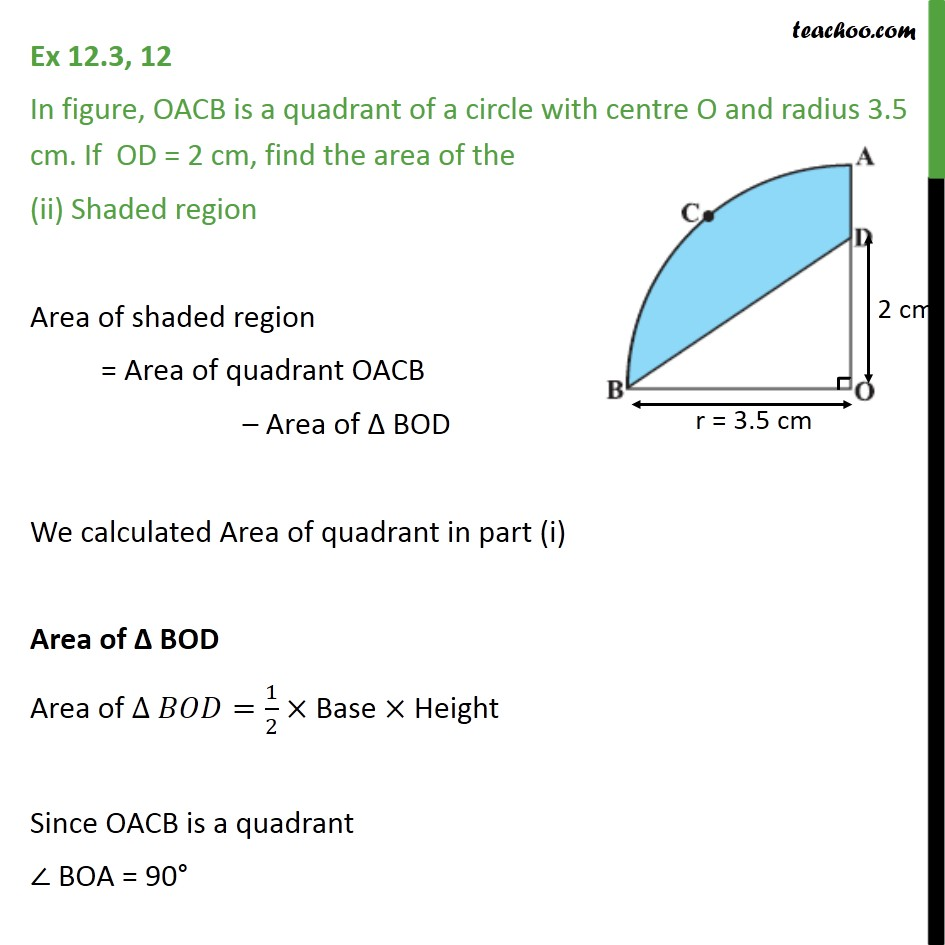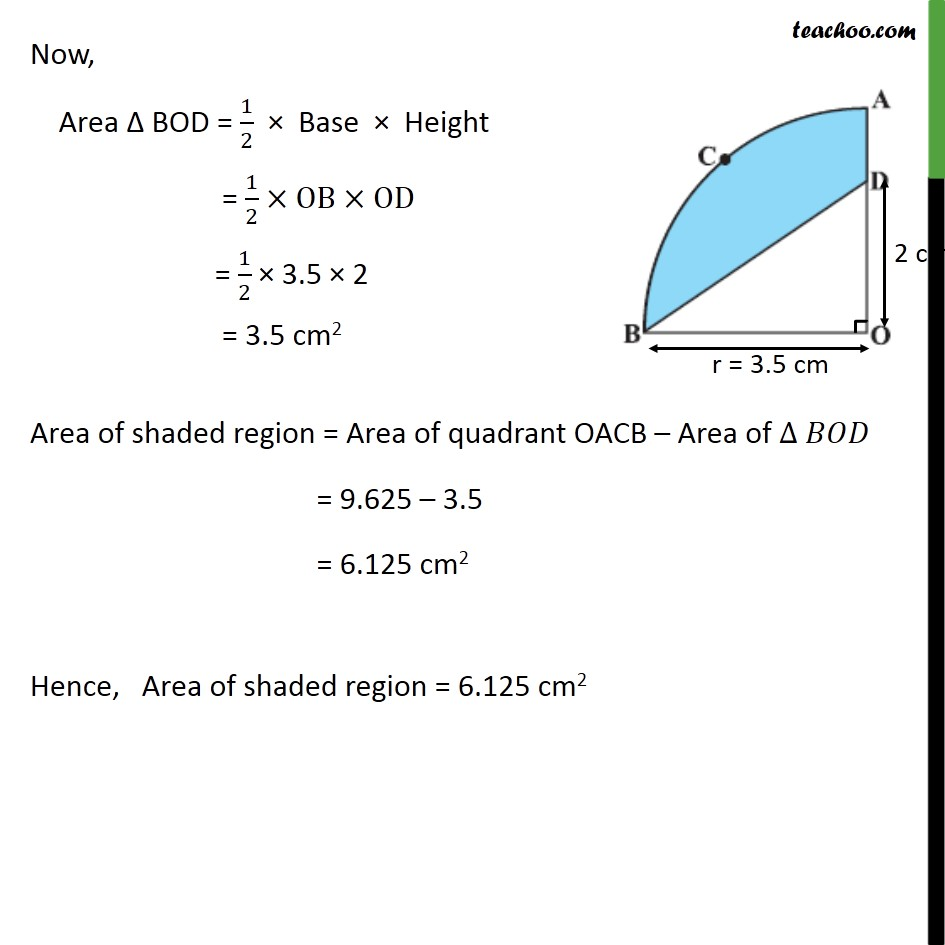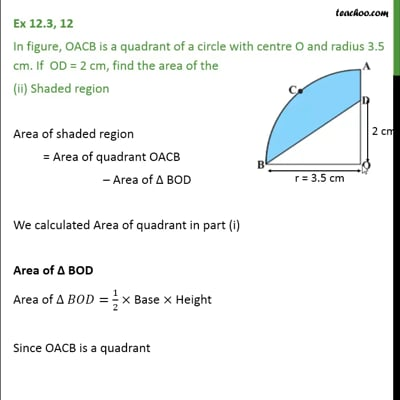This video is only available for Teachoo black users

Solve all your doubts with Teachoo Black (new monthly pack available now!)

### Transcript

Ex 12.3, 12 In figure, OACB is a quadrant of a circle with centre O and radius 3.5 cm. If OD = 2 cm, find the area of the quadrant OACB, It is given that, OACB is a quadrant Given radius = 3.5 cm We know that Area of Quadrant OACB = 1/4 Area of a circle Area of quadrant OACB = 1/4 2 = 1/4 22/7 (3.5)2 = 1/4 22/7 3.5 3.5 = 3.625 cm2 Ex 12.3, 12 In figure, OACB is a quadrant of a circle with centre O and radius 3.5 cm. If OD = 2 cm, find the area of the (ii) Shaded region Area of shaded region = Area of quadrant OACB Area of BOD We calculated Area of quadrant in part (i) Area of BOD Area of =1/2 Base Height Since OACB is a quadrant BOA = 90 Now, Area BOD = 1/2 Base Height = 1/2 OB OD = 1/2 3.5 2 = 3.5 cm2 Area of shaded region = Area of quadrant OACB Area of = 9.625 3.5 = 6.125 cm2 Hence, Area of shaded region = 6.125 cm2